# Cost-Volume-Profit Analysis

﻿
Cost-Volume-Profit Analysis

Cost-Volume-profit (CVP), in managerial economics is a form of cost accounting. It is a simplified model, useful for elementary instruction and for short-run decisions.

Cost-volume-profit (CVP) analysis expands the use of information provided by breakeven analysis. A critical part of CVP analysis is the point where total revenues equal total costs (both fixed and variable costs). At this breakeven point (BEP), a company will experience no income or loss. This BEP can be an initial examination that precedes more detailed CVP analysis.

Cost-volume-profit analysis employs the same basic assumptions as in breakeven analysis. The assumptions underlying CVP analysis are:

The behavior of both costs and revenues is linear throughout the relevant range of activity. (This assumption precludes the concept of volume discounts on either purchased materials or sales.) Costs can be classified accurately as either fixed or variable. Changes in activity are the only factors that affect costs. All units produced are sold (there is no ending finished goods inventory). When a company sells more than one type of product, the sales mix (the ratio of each product to total sales) will remain constant.

The components of Cost-Volume-Profit Analysis are:

• Level or volume of activity
• Unit Selling Prices
• Variable cost per unit
• Total fixed costs
• Sales mix

## Assumptions

CVP assumes the following:

• Constant sales price;
• Constant variable cost per unit;
• Constant total fixed cost;
• Constant sales mix;
• Units sold equal units produced.

These are simplifying, largely linearizing assumptions, which are often implicitly assumed in elementary discussions of costs and profits. In more advanced treatments and practice, costs and revenue are nonlinear and the analysis is more complicated, but the intuition afforded by linear CVP remains basic and useful.

One of the main Methods of calculating CVP is Profit volume ratio: which is (contribution /sales)*100 = this gives us profit volume ratio.

• contribution stands for Sales minus variable costs.

Therefore it gives us the profit added per unit of variable costs.

## Model

### Basic graph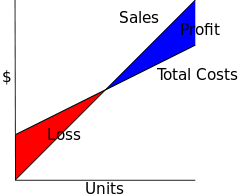Basic graph of CVP, demonstrating relation of Total Costs, Sales, and Profit and Loss.

The assumptions of the CVP model yield the following linear equations for total costs and total revenue (sales):$\text{Total Costs} = \text{Fixed Costs} + (\text{Unit Variable Cost} \times \text{Number of Units}$)$\text{Total Revenue} = \text{Sales Price} \times \text{Number of Units}$

These are linear because of the assumptions of constant costs and prices, and there is no distinction between Units Produced and Units Sold, as these are assumed to be equal. Note that when such a chart is drawn, the linear CVP model is assumed, often implicitly.

In symbols:$\text{TC} = \text{TFC} + \text{V} \times \text{X}$$\text{TR} = \text{P} \times \text{X}$

where

• TC = Total Costs
• TFC = Total Fixed Costs
• V = Unit Variable Cost (Variable Cost per Unit)
• X = Number of Units
• TR = S = Total Revenue = Sales
• P = (Unit) Sales Price

Profit is computed as TR-TC; it is a profit if positive, a loss if negative.

### Break down

Costs and Sales can be broken down, which provide further insight into operations.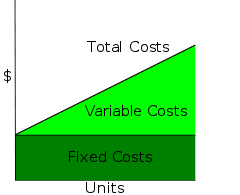Decomposing Total Costs as Fixed Costs plus Variable Costs.

One can decompose Total Costs as Fixed Costs plus Variable Costs:$\text{TC} = \text{TFC} + \text{V} \times \text{X}$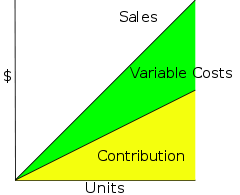Decomposing Sales as Contribution plus Variable Costs.

Following a matching principle of matching a portion of sales against variable costs, one can decompose Sales as Contribution plus Variable Costs, where contribution is "what's left after deducting variable costs". One can think of contribution as "the marginal contribution of a unit to the profit", or "contribution towards offsetting fixed costs".

In symbols:\begin{align} \text{TR} &= \text{P} \times \text{X}\\ &= \bigl(\left(\text{P} - \text{V} \right)+\text{V}\bigr)\times \text{X}\\ &= \left(\text{C}+\text{V}\right)\times \text{X}\\ &= \text{C}\times\text{X} + \text{V}\times \text{X} \end{align}

where

• C = Unit Contribution (Margin)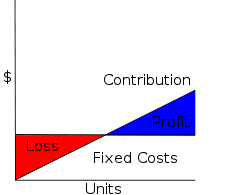Profit and Loss as Contribution minus Fixed Costs.

Subtracting Variable Costs from both Costs and Sales yields the simplified diagram and equation for Profit and Loss.

In symbols:\begin{align} \text{PL} &= \text{TR} - \text{TC}\\ &= \left(\text{C}+\text{V}\right)\times \text{X} - \left(\text{TFC} + \text{V} \times \text{X}\right)\\ &= \text{C} \times \text{X} - \text{TFC} \end{align}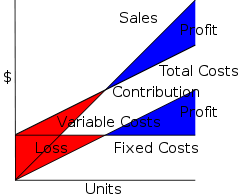Diagram relating all quantities in CVP.

These diagrams can be related by a rather busy diagram, which demonstrates how if one subtracts Variable Costs, the Sales and Total Costs lines shift down to become the Contribution and Fixed Costs lines. Note that the Profit and Loss for any given number of unit sales is the same, and in particular the break-even point is the same, whether one computes by Sales = Total Costs or as Contribution = Fixed Costs. Mathematically, the contribution graph is obtained from the sales graph by a shear, to be precise$\left(\begin{smallmatrix}1 & 0\\ -V & 1\end{smallmatrix}\right)$, where V are Unit Variable Costs.

## Applications

CVP simplifies the computation of breakeven in break even analysis, and more generally allows simple computation of Target Income Sales. It simplifies analysis of short run trade-offs in operational decisions.

## Limitations

CVP is a short run, marginal analysis: it assumes that unit variable costs and unit revenues are constant, which is appropriate for small deviations from current production and sales, and assumes a neat division between fixed costs and variable costs, though in the long run all costs are variable. For longer-term analysis that considers the entire life-cycle of a product, one therefore often prefers activity-based costing or throughput accounting.

Wikimedia Foundation. 2010.

### Look at other dictionaries:

• Cost-Volume Profit Analysis — A method of cost accounting used in managerial economics. Cost volume profit analysis is based upon determining the breakeven point of cost and volume of goods. It can be useful for managers making short term economic decisions, and also for… …   Investment dictionary

• cost-volume-profit analysis — See: breakeven analysis …   Accounting dictionary

• Cost accounting — Accountancy Key concepts Accountant · Accounting period · Bookkeeping · Cash and accrual basis · Cash flow management · Chart of accounts  …   Wikipedia

• Profit-Volume (PV) Chart — A graphic that shows the relationship between a company s earnings (or losses) and its sales. The chart tells how different levels of sales affect a company s profits. Companies can use profit volume charts to establish sales goals, to analyze… …   Investment dictionary

• Cost to serve — is a process driven accountancy tool to calculate the profitability of a customer account, based on the actual business activities and overhead costs incurred to service that customer. In the context of supply chain management it can be used… …   Wikipedia

• breakeven analysis — cost volume profit analysis; = CVP analysis The technique used in management accounting in which costs are analysed according to cost behaviour characteristics into fixed costs and variable costs and compared to sales revenue in order to… …   Accounting dictionary

• breakeven analysis — cost volume profit analysis; CVP analysis The technique used in management accounting in which costs are analysed according to cost behaviour characteristics into fixed costs and variable costs and compared to sales revenue in order to determine… …   Big dictionary of business and management

• Marginal cost — A typical marginal cost curve with marginal revenue overlaid In economics and finance, marginal cost is the change in total cost that arises when the quantity produced changes by one unit. That is, it is the cost of producing one more unit of a… …   Wikipedia

• Total cost — In economics, and cost accounting, total cost (or total costs) describes the total economic cost of production and is made up of variable costs, which vary according to quantity produced such as raw materials, plus fixed costs, which are… …   Wikipedia

• Fixed cost — Fixed costs are business expenses that are not dependent on the level of production or sales. They tend to be time related, such as salaries or rents being paid per month . This is in contrast to Variable costs, which are volume related (and are… …   Wikipedia